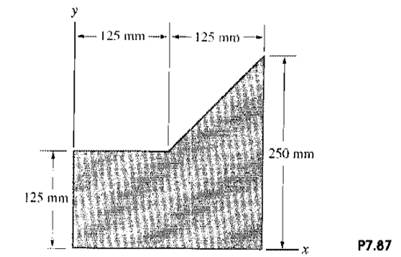### Create an Account

Home / Questions / Determine the mass moment of inertia of the plate in Problem 787 about the y axis Problem...

# Determine the mass moment of inertia of the plate in Problem 787 about the y axis Problem 787 The homogeneous thin plate weighs 50 N Determine its mass moment of inertia about the x axis

Determine the mass moment of inertia of the plate in Problem 7.87 about the y axis.

Problem 7.87

The homogeneous, thin plate weighs 50 N. Determine its mass moment of inertia about the x axis.Jun 15 2020 View more View LessSubscribe To Get Solution